# Surging 10,000% in three and a half days? How is Fraxlend’s interest rate calculated?

## How does Fraxlend calculate its interest rate when it surges 10,000% in just three and a half days?

Why did Curve founder prioritize repaying Fraxlend debt?

As the personal debt risk of Curve founder Michael Egorov escalated, there has been increasing discussion surrounding the issue of Fraxlend rates.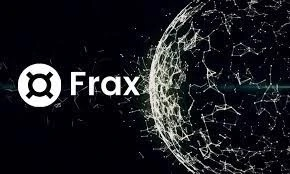The reason for this is that Fraxlend adopts a relatively unique rate adjustment mechanism, which has resulted in the loan interest rate in the CRV/FRAX market of Fraxlend rapidly rising from yesterday until today, “forcing” Egorov to repay a debt of \$7.13 million on the platform this afternoon.

So, how are Fraxlend rates calculated? Why has this number risen so quickly? Combining the official documentation of Frax Finance and the tweets from its developer Drake Evans, we have briefly summarized the algorithm.

Firstly, according to the documentation of Frax Finance, Fraxlend adopts three different rate variation algorithms for different markets: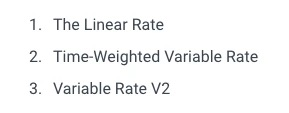• Linear rate: Simply put, it is a basic algorithm where the rate increases linearly with market utilization.

• Time-weighted variable rate: An algorithm that determines rate increase or decrease based on market utilization and adjusts the rate at fixed time intervals.

• Variable rate V2: A composite algorithm that combines the previous two, using a linear rate function to determine the current rate, but also adjusts the maximum rate using the formula of time-weighted variable rate.

Okay, you might find this explanation even more confusing, but don’t worry, let’s continue.

According to Drake’s explanation, the CRV/FRAX market of Fraxlend adopts the second algorithm (i.e. time-weighted variable rate), and the mechanism of this algorithm is as follows:

• Firstly, Fraxlend sets a target utilization rate range for a specific market. When the market utilization rate is within this range, the rate does not need to change.

• When the market utilization rate is below the target range, the rate gradually decreases at a certain multiplier at fixed time intervals, until it reaches the minimum rate.

• When the market utilization rate is above the target range, the rate gradually increases at a certain multiplier at fixed time intervals, until it reaches the maximum rate.

This specific time interval is called the half-life in the design of Fraxlend, referring to the time required for the rate to halve when the market utilization rate is 0, as well as the time required for the rate to double when the market utilization rate is 0.

According to the contract of the CRV/FRAX market, the target utilization rate range is 75%-85%, the minimum rate is 0.5%, the maximum rate is 10000%, and the half-life is 12 hours.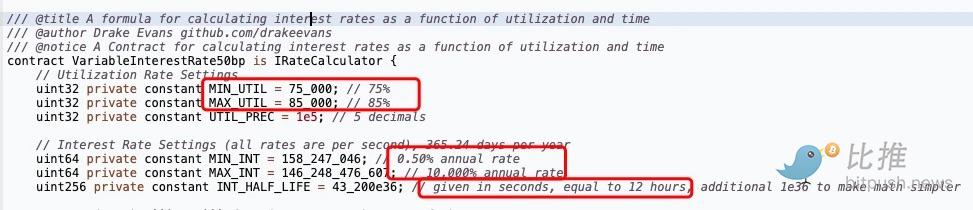This also means that for the CRV/FRAX market:

• When the market utilization rate is between 75% and 85%, the interest rate does not need to change.

• When the market utilization rate is below 75%, the interest rate will gradually decrease at a certain “multiplier” every 12 hours until it reaches 0.5%.

• When the market utilization rate is above 85%, the interest rate will gradually increase at a certain “multiplier” every 12 hours until it reaches 10000%.

Therefore, for a considerable period of time from yesterday to today, due to the continuous high utilization rate of the CRV/FRAX market in Fraxlend, even close to 100%, the lending interest rate of the pool has continued to rise.

However, this does not mean that the interest rate of this market will double every 12 hours as some rumors say, and it will skyrocket to 10000% in three and a half days (this statement comes from Delphi Digital, but Delphi Digital only listed the case of 100% utilization rate and did not say it is a general situation after exceeding 85%), because in reality, the utilization rate of this market did not continuously stay at 100% (which is not possible), so the “multiplier” mentioned earlier is not always doubled.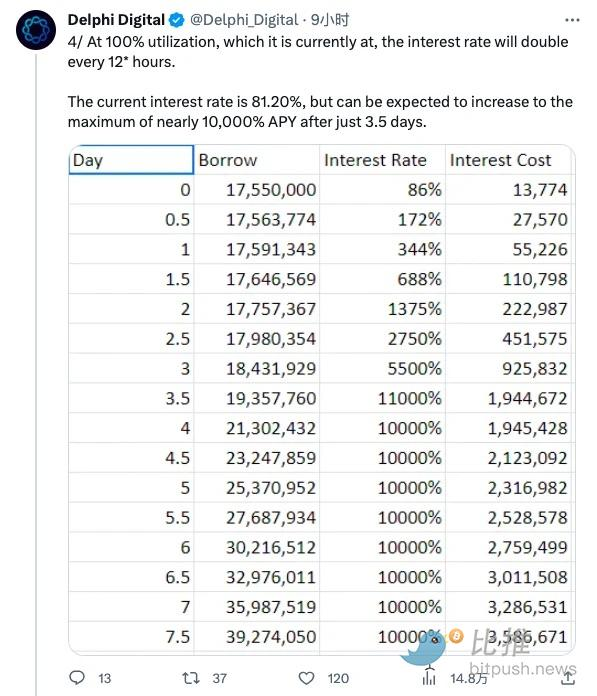According to Drake’s additional introduction, after the utilization rate of the CRV/FRAX market exceeds 85%, the multiplier for the growth of the interest rate varies at different levels of utilization rate.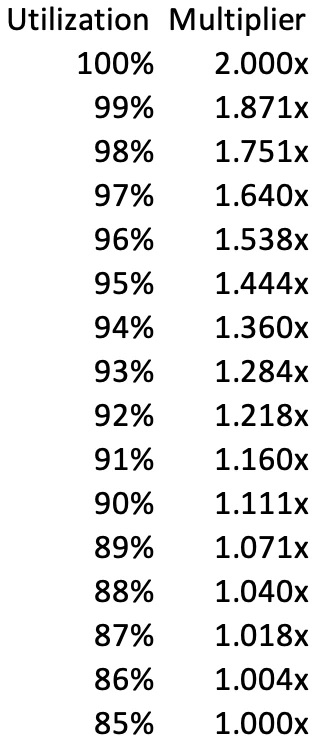As shown in the above figure, when the market utilization rate is 85%, the multiplier is 1, which means that the interest rate will remain unchanged; but as the utilization rate increases, the multiplier will gradually increase; until the utilization rate reaches 100%, the multiplier will become 2, which means that the doubling growth as mentioned by Delphi Digital will begin.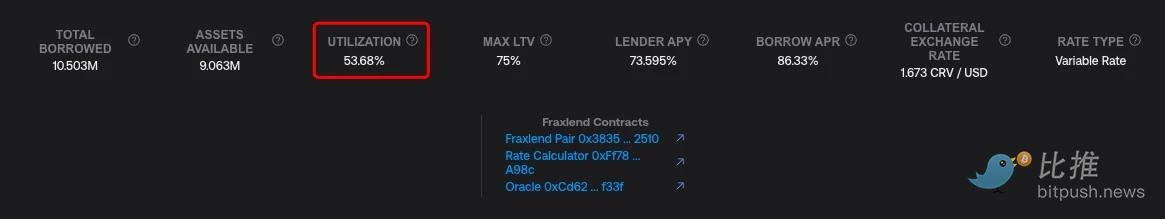Currently, with Egorov’s repayment, the utilization rate of the CRV/FRAX market has dropped to 53.68%, which means that if this situation can be maintained, the interest rate will further decrease after 12 hours. In this way, Egorov’s pressure will also be significantly reduced.

So it is not difficult to understand why Egorov paid off part of the debt of Fraxlend in advance. At least, the utilization rate needs to be suppressed first, otherwise, it would be difficult to withstand the continuous increase in interest rates.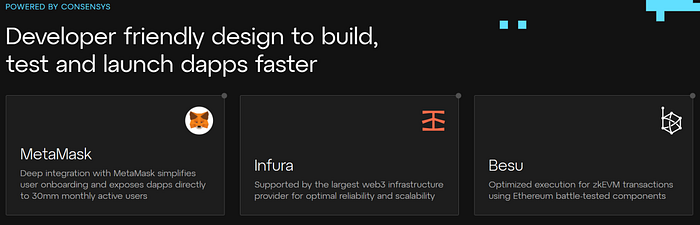##### Linea With the strong endorsement of Consen...##### Evening Must-Read | Challenges and Opportun...##### Overnight, it multiplied by a thousand time...##### Understanding the whole story of the Curve ...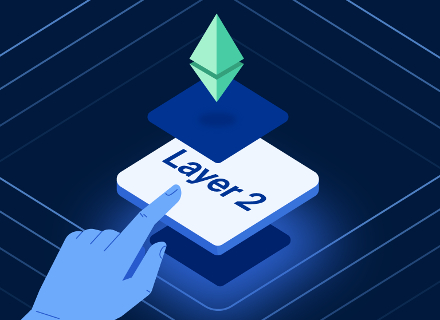##### Conversation with Coinbase Senior Director ...##### Curve Pool Reentry Vulnerability Exploitati...#### Blockchain

Discover the transformative power of blockchain technology and its potential to revolutionize industries, enhance security, and foster decentralized trust.